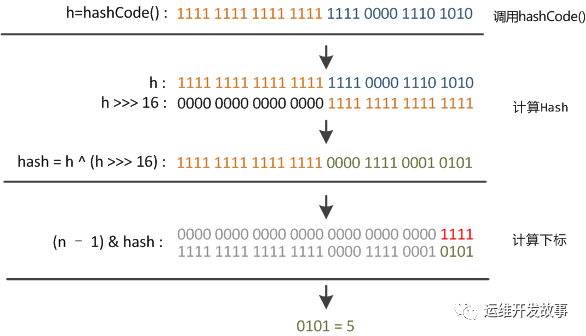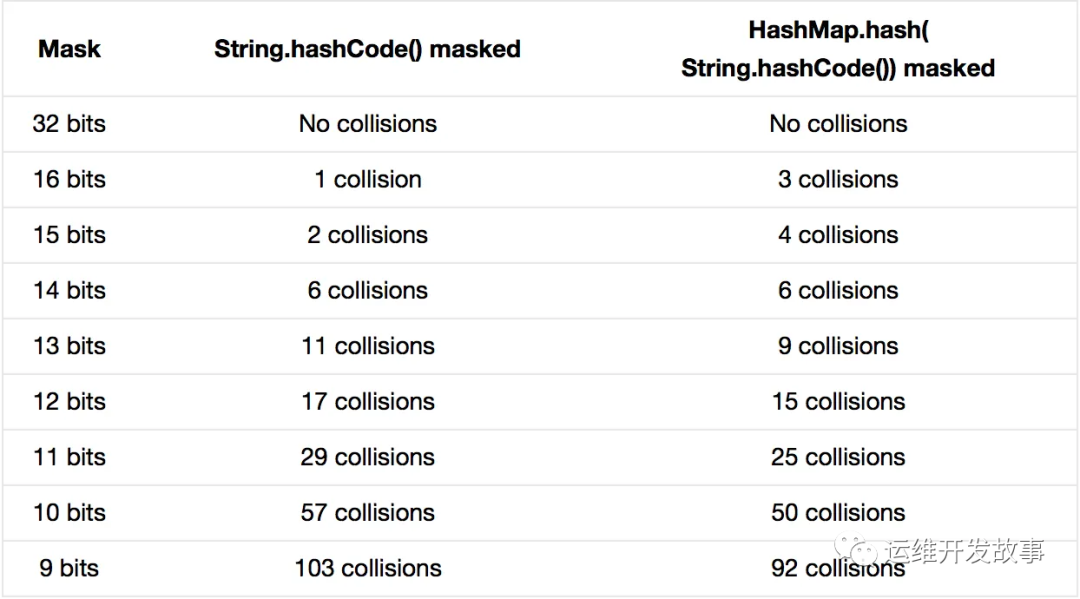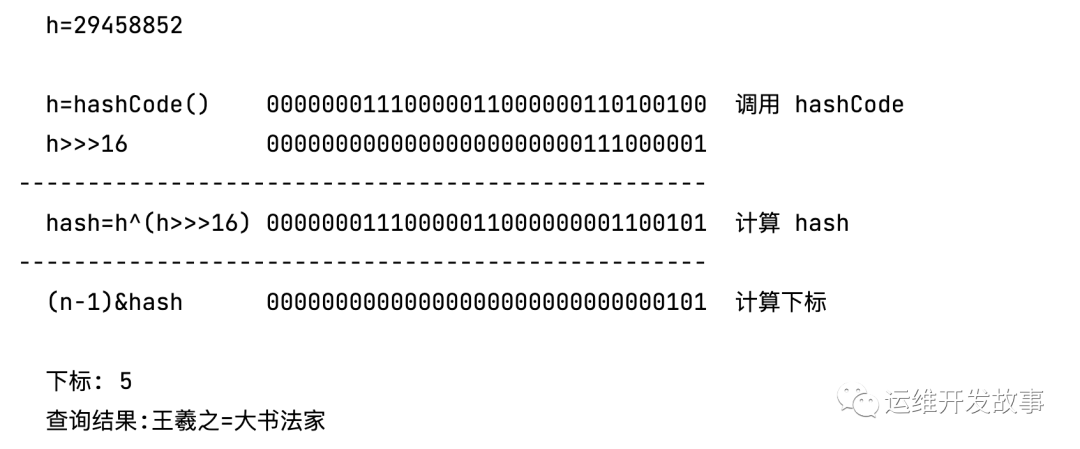# HashMap 计算 Hash 值的扰动函数

0收藏

### 计算过程

``````//java 8 中的散列值优化函数
static final int hash(Object key) {
int h;
return (key == null) ? 0 : (h = key.hashCode()) ^ (h >>> 16);
}``````

``````// n = hashmap 的长度
p = tab[i = (n - 1) & hash])``````

``````10000000 00100000 00001001
&   00000000 00000000 00001111
------------------------------
00000000 00000000 00001001  // 高位全部归 0, 只保留后四位``````### 代码演示‍

``````import java.lang.reflect.Field;
import java.util.HashMap;

/**
* HashMap 计算 hashKey
* <p>
* 演示：扰动函数
*
* @see HashMap#hash(Object)
*/
public class HashKeyTest {

public static void main(String[] args) throws NoSuchFieldException, IllegalAccessException {
HashMap<String, String> map = new HashMap<>();
String k = "王羲之";
String v = "大书法家";
map.put(k, v);

Field field = map.getClass().getDeclaredField("table");
field.setAccessible(Boolean.TRUE);
Object[] nodes = (Object[]) field.get(map);

int h = k.hashCode();
System.out.println("  h=" + h);
System.out.println();
// 调用 hashCode 结果
System.out.println("  h=hashCode()    " + num0x(h) + "  调用 hashCode");
// 无符号右移 16
System.out.println("  h>>>16          " + num0x(h >>> 16));
System.out.println("--------------------------------------------------");
// 计算 hash
System.out.println("  hash=h^(h>>>16) " + num0x(h ^ (h >>> 16)) + "  计算 hash");
System.out.println("--------------------------------------------------");
// 计算下标
System.out.println("  (n-1)&hash      " + num0x(15 & (h ^ (h >>> 16))) + "  计算下标");
System.out.println();
int idx = (15 & (h ^ (h >>> 16)));
// 输出下标
System.out.println("  下标: " + idx);
// 在下标中去获取数据
System.out.println("  查询结果:" + nodes[idx]);
}

/**
* 输入 int 转换为 二进制字符串
*
* @param num 数字
* @return 二进制字符串
*/
static String num0x(int num) {
String num0x = "";
for (int i = 31; i >= 0; i--) {
num0x += (num & 1 << i) == 0 ? "0" : "1";
}
return num0x;
}
}``````相关推荐
帖子
视频
声望
粉丝
社区精华内容